# Index construction Compression of postings Paolo Ferragina Dipartimento

• Slides: 15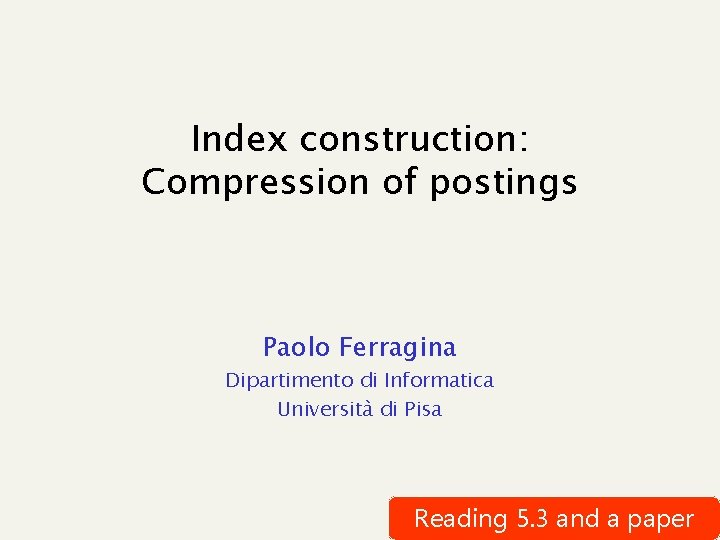Index construction: Compression of postings Paolo Ferragina Dipartimento di Informatica Università di Pisa Reading 5. 3 and a paper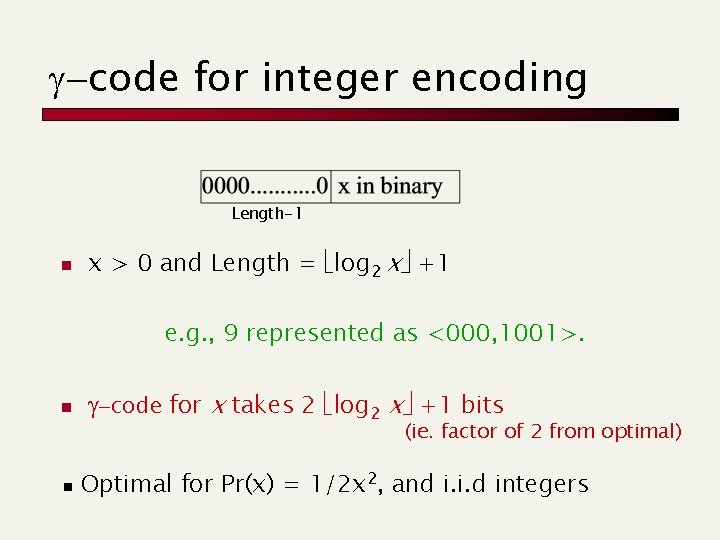g-code for integer encoding Length-1 n x > 0 and Length = log 2 x +1 e. g. , 9 represented as <000, 1001>. n n g-code for x takes 2 log 2 x +1 bits (ie. factor of 2 from optimal) Optimal for Pr(x) = 1/2 x 2, and i. i. d integers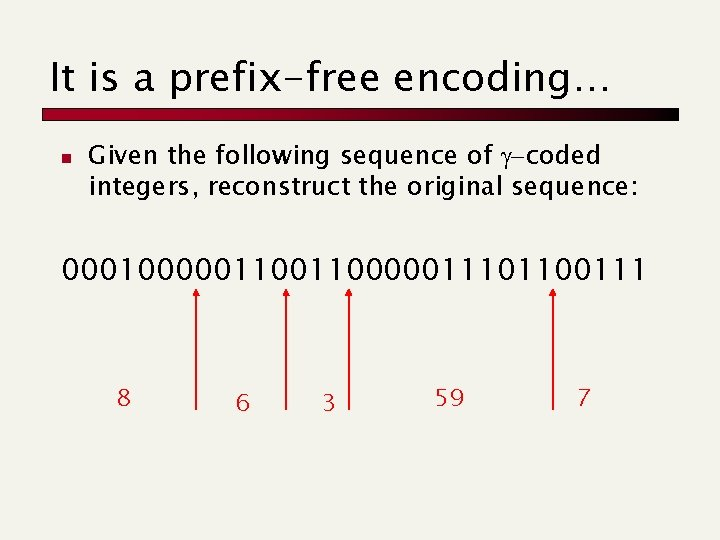It is a prefix-free encoding… n Given the following sequence of g-coded integers, reconstruct the original sequence: 000100000110000011101100111 8 6 3 59 7d-code for integer encoding n n Use g-coding to reduce the length of the first field Useful for medium-sized integers e. g. , 19 represented as <00, 101, 10011>. n n d-coding x takes about log 2 x + 2 log 2( log 2 x ) + 2 bits. Optimal for Pr(x) = 1/2 x(log x)2, and i. i. d integers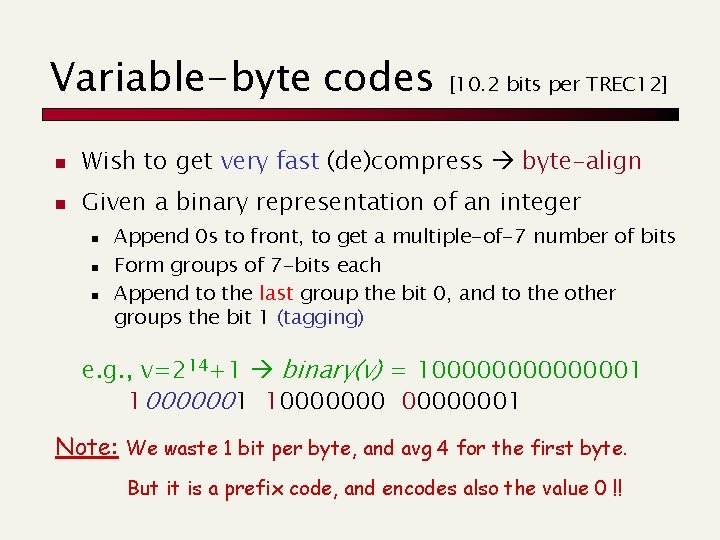Variable-byte codes [10. 2 bits per TREC 12] n Wish to get very fast (de)compress byte-align n Given a binary representation of an integer n n n Append 0 s to front, to get a multiple-of-7 number of bits Form groups of 7 -bits each Append to the last group the bit 0, and to the other groups the bit 1 (tagging) e. g. , v=214+1 binary(v) = 100000001 100000001 Note: We waste 1 bit per byte, and avg 4 for the first byte. But it is a prefix code, and encodes also the value 0 !!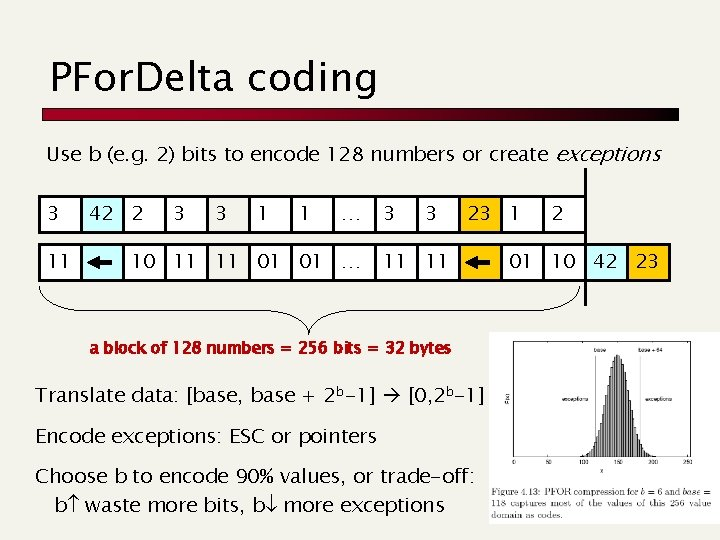PFor. Delta coding Use b (e. g. 2) bits to encode 128 numbers or create exceptions 3 11 42 2 3 3 1 1 … 10 11 11 01 01 … 3 3 23 1 11 11 a block of 128 numbers = 256 bits = 32 bytes Translate data: [base, base + 2 b-1] [0, 2 b-1] Encode exceptions: ESC or pointers Choose b to encode 90% values, or trade-off: b waste more bits, b more exceptions 2 01 10 42 23Random access to postings lists and other data types (e. g. encoding skips? ) Paolo Ferragina Dipartimento di Informatica Università di Pisa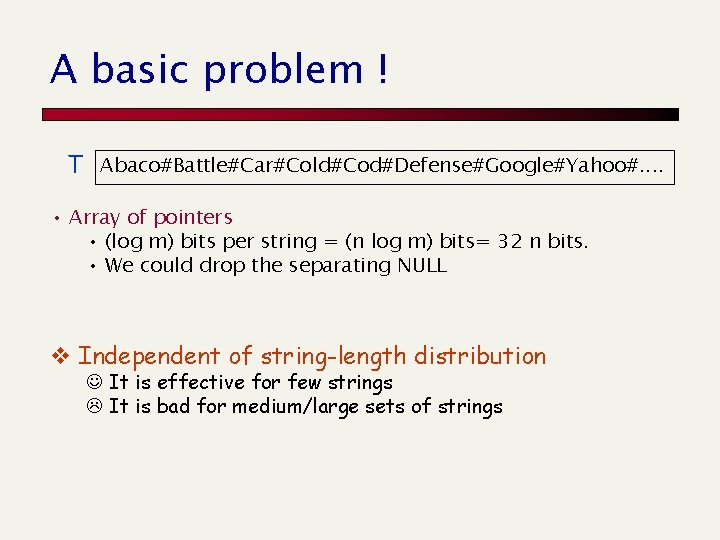A basic problem ! T Abaco#Battle#Car#Cold#Cod#Defense#Google#Yahoo#. . • Array of pointers • (log m) bits per string = (n log m) bits= 32 n bits. • We could drop the separating NULL v Independent of string-length distribution J It is effective for few strings L It is bad for medium/large sets of strings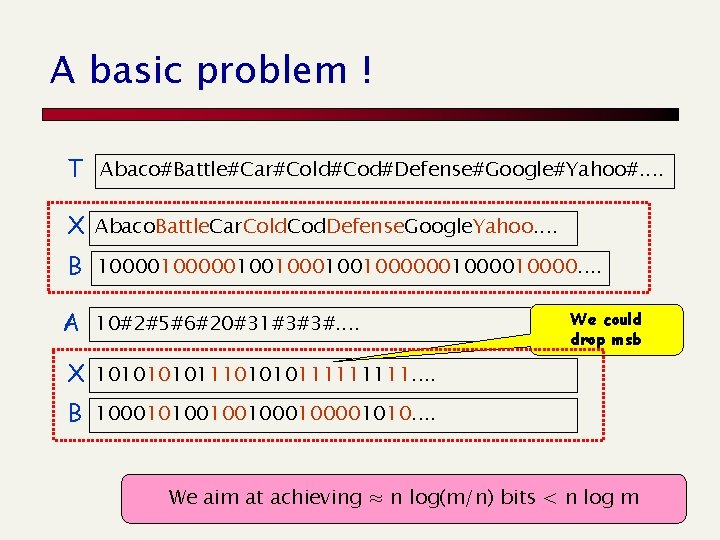A basic problem ! T Abaco#Battle#Car#Cold#Cod#Defense#Google#Yahoo#. . X Abaco. Battle. Car. Cold. Cod. Defense. Google. Yahoo. . B 100000100100000010000. . A 10#2#5#6#20#31#3#3#. . We could drop msb X 10101110101011111. . B 100010100100100001010. . We aim at achieving ≈ n log(m/n) bits < n log m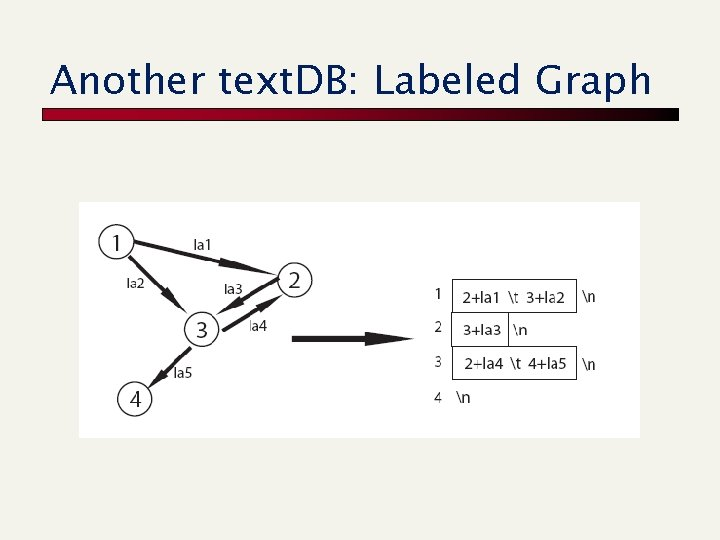Another text. DB: Labeled GraphRank/Select Wish to index the bit vector B compressed. Select 1(3) = 8 B 001010101011111110000011010101. . Rank 1(6) = 2 • Rankb(i) = number of b in B[1, i] m = |B| n = #1 • Selectb(i) = position of the i-th b in B Do exist data structures that solve this problem in O(1) query time and efficient space (i. e. +o(m) bits additional)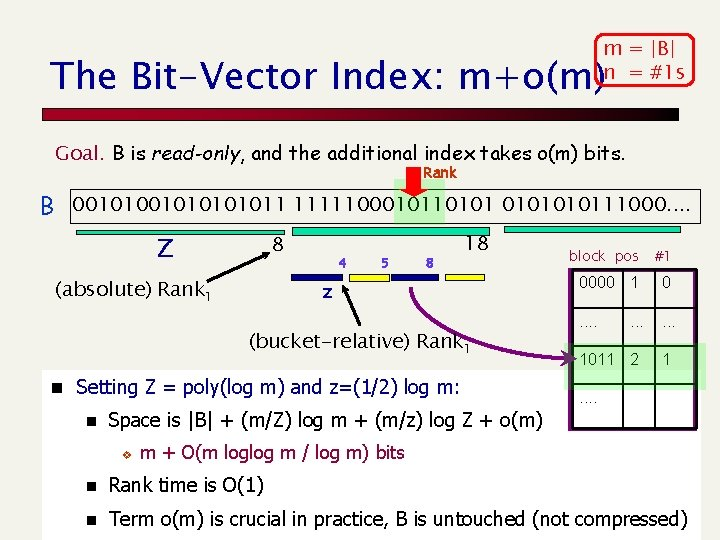m = |B| n = #1 s The Bit-Vector Index: m+o(m) Goal. B is read-only, and the additional index takes o(m) bits. Rank B 001010101011 111110001011010111000. . 8 Z (absolute) Rank 1 4 5 8 18 z (bucket-relative) Rank 1 n Setting Z = poly(log m) and z=(1/2) log m: n block pos #1 0000 1 0 . . 1011 2 1 . . Space is |B| + (m/Z) log m + (m/z) log Z + o(m) v m + O(m loglog m / log m) bits n Rank time is O(1) n Term o(m) is crucial in practice, B is untouched (not compressed)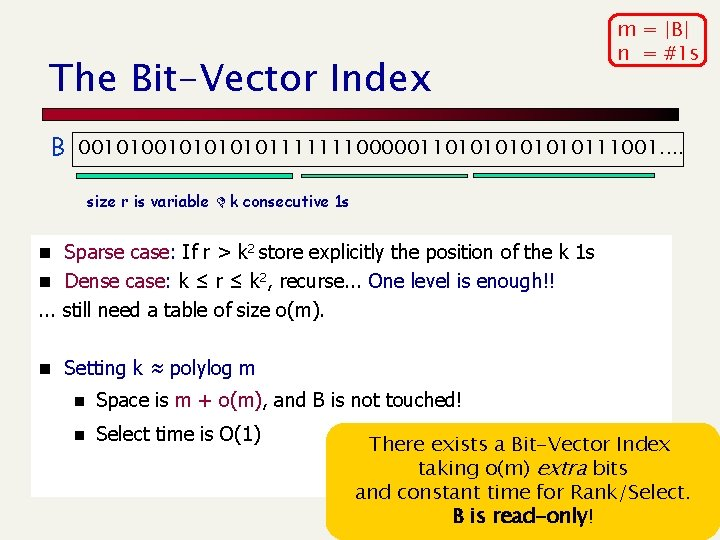The Bit-Vector Index m = |B| n = #1 s B 00101010101111111000001101010111001. . size r is variable k consecutive 1 s n Sparse case: If r > k 2 store explicitly the position of the k 1 s n Dense case: k ≤ r ≤ k 2, recurse. . . One level is enough!! . . . still need a table of size o(m). n Setting k ≈ polylog m n Space is m + o(m), and B is not touched! n Select time is O(1) There exists a Bit-Vector Index taking o(m) extra bits and constant time for Rank/Select. B is read-only!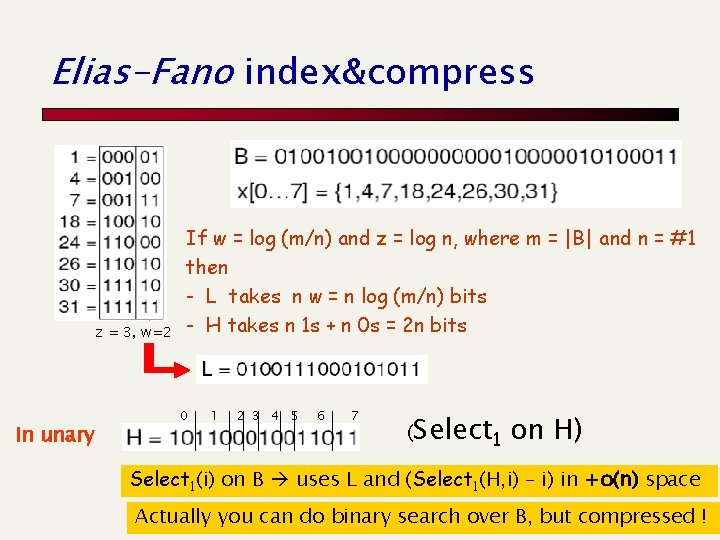Elias-Fano index&compress z = 3, w=2 In unary If w = log (m/n) and z = log n, where m = |B| and n = #1 then - L takes n w = n log (m/n) bits - H takes n 1 s + n 0 s = 2 n bits 0 1 2 3 4 5 6 7 (Select 1 on H) Select 1(i) on B uses L and (Select 1(H, i) – i) in +o(n) space Actually you can do binary search over B, but compressed !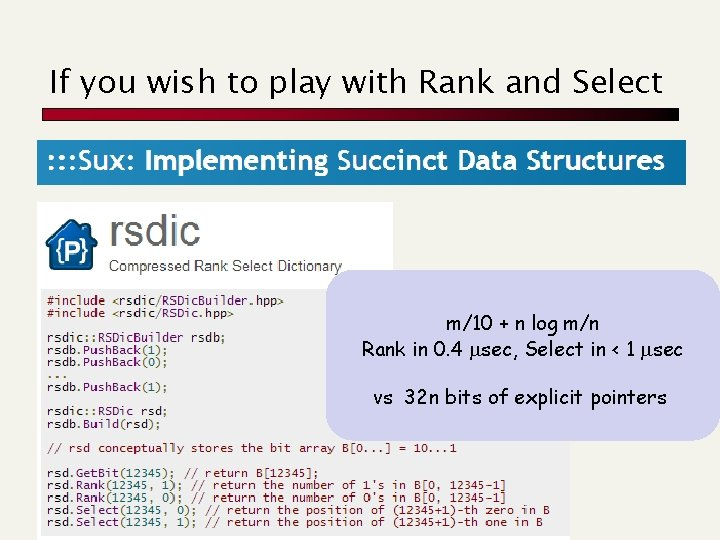If you wish to play with Rank and Select m/10 + n log m/n Rank in 0. 4 msec, Select in < 1 msec vs 32 n bits of explicit pointers